Electron. J. Diff. Equ., Vol. 2010(2010), No. 99, pp. 1-5.

### Stability of delay differential equations with oscillating coefficients Michael I. Gil'

Abstract:
We study the solutions to the delay differential equation equation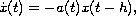where the coefficient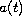is not necessarily positive. It is proved that this equation is exponentially stable provided that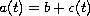for some positive constant b less than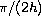, and the integral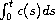is sufficiently small for all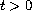. In this case the 3/2-stability theorem is improved.

Submitted April 13, 2010. Published July 22, 2010.
Math Subject Classifications: 34K20.
Key Words: Linear delay differential equation; exponential stability.

Show me the PDF file (171 KB), TEX file, and other files for this article.Michael I. Gil' Department of Mathematics Ben Gurion University of the Negev P.0. Box 653, Beer-Sheva 84105, Israel email: gilmi@cs.bgu.ac.il